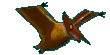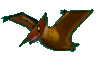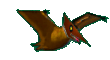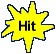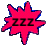Math Help - Arithmetic Fun - Percentages 1% to 15% - Game Tips:

- Ten percent means 'one tenth of the amount'. Therefore 10% of \$400 = \$40.
- One percent means 'one hundredth of the amount'. Therefore 1% of \$400 = \$4.

- Once you know one percent, you can find any percentage as a multiple of one percent.
- 2% of \$400 is the same as 2 times (1% of \$400) = 2 times \$4 = \$8.
- 6% of \$400 is the same as 6 times (1% of \$400) = 6 times \$4 = \$24.
- 15% of \$400 is the same as 15 times (1% of \$400) = 15 times \$4 = \$60.

- Your Game Score is reduced by the number of pterodactyl hits.

- To slow the game speed repeat tap/click on the word Slider.
- To increase the game speed repeat tap/click on the word Math.
- Speed can also be adjusted with a keyboard's - and + keys.

- Refresh/Reload the web page to restart the game.